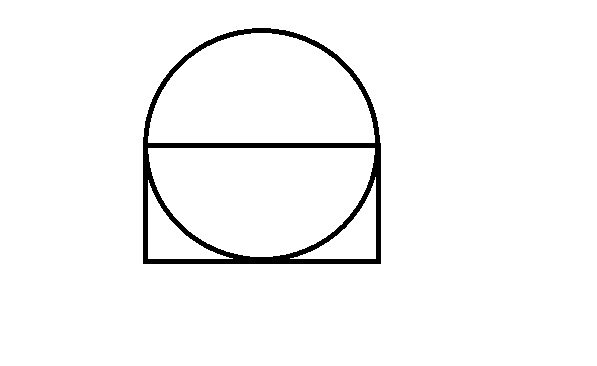# Mensuration Questions for SBI-Clerk

0
330

## Mensuration Questions for SBI-Clerk

Download SBI Clerk Mensuration  Questions & Answers PDF for SBI Clerk Prelims and Mains exam. Very Important SBI Clerk Mensuration Questions with solutions.

Question 1: The area of a square is 1444 square metres. The breadth of a rectangle is 1/4th the side of the square and the length of the rectangle is thrice the breadth. What is the difference between the area of the square and the area of the rectangle?

a) 1152.38 sq.mtr.

b) 1169.33 sq.mtr.

c) 1181.21 sq.mtr.

d) 1173.25 sq.mtr.

e) None of these.

Question 2: The area of a square is the same as that of a circle. What is the ratio of the side of the square to the radius of the circle?

a) 1:pi

b) 1: sq.root pi

c) sq.root pi : 1

d) pi square: 1

e) None of the above

Question 3: The area of a rectangle is twice the area of a triangle. The perimeter of the rectangle is 58cm. What is the area of the triangle?

a) 106 $cm^{2}$

b) 108 $cm^{2}$

c) 104 $cm^{2}$

d) Cannot be determined

e) None of these

Question 4: The area of a triangle is half the area of a square. The perimeter of the square is 224cms What is the area of the triangle?

a) 1856 $cm^2$

b) 1658 $cm^2$

c) 1558 $cm^2$

d) 1586 $cm^2$

e) None of these

Question 5: Area of the circle is 616 $cm^{2}$. What is the area of the rectangle? (One side of the rectangle passes through the center of the circle)a) 786 $cm^{2}$

b) 196 $cm^{2}$

c) 392 $cm^{2}$

d) Cannot be determined

e) None of these

Question 6: The area of a square is thrice the area of a rectangle If the area of the square is 225 sq cm and the length of the rectangle is 15 cm what is the difference between the breadth of the rectangle and the side of the square?

a) 8 cm

b) 10 cm

c) 12 cm

d) 6 cm

e) None of these

Question 7: The respective ratio of curved surface area and total surface area of a cylinder is 4:5. If the curved surface area of the cylinder is 1232 cm2, what is the height? (in cm)

a) 392 cm

b) 394 cm

c) 396 cm

d) 300 cm

e) None of these

Question 8: The perimeter of a rectangle whose length is 6rn more than its breadth is 84 m. What would be the area of a triangle whose base is equal to the diagonal of the rectangle and whose height is equal to the length of the rectangle? (in m )

a) 324

b) 372

c) 360

d) 364

e) 348

Question 9: The area of a circle is equal to the area of a rectangle with perimeter equal to 42 metres and breadth equal to 8.5 metre What is the area of the circle?

a) 116.25 sq.mts

b) 104.25 sq.mts

c) 146.25 sq.mts

d) 128.25 sq.rnts

e) None of these

Question 10: The area of a circle is equal to the area of a rectangle with perimeter equal to 35 metres and breadth equal to 5.5 metres. What is the area of the circle?

a) 88 sq.mts.

b) 72 sq.mts.

c) 66 sq.mts.

d) 54 sq.mts.

e) one of these

Side = $\sqrt{1444}$ = 38
Breadth of rectangle = $\frac{1}{4}$th of 38 = 9.5
Length = thrice of breadth = 28.5
Area of rectangle =  9.5 x 28.5 = 270.75
Difference = 1173.25 m2

$S^{2} = pi * r^{2}$. So, s/r = $\sqrt \pi$

The data provided in question is insufficient for calculation.

Hence, we cannot calculate the required area of the triangle.

Let’s assume that the side of the square is s.

Now, Perimeter 4s=224, hence, s=56.

Area of triangle = .5(area of square) = .5*(562) = 1568 which is not any of the option.

Area of the circle =$\pi d^2/4$ = 616
d = 28
The length of the rectangle is equal to diameter which is 28 and breadth is equal to radius which is 114
Area = 28*14 = 392

area of square = $(side)^2$ = 225

Side of square = 15 cm

It is given that area of square is thrice area of rectangle ,so

225 = 3 x L x B where L and B are length and breadth of rectangle.

Given that length of rectangle is 15 cm so B = 5 cm

Side of square – Breadth of rectangle = 15 -5 =10 cm

Let R and H be the radius and height of cylinder

Curved Surface area of cylinder = 2$\barwedge$RH = 1232

($\barwedge$RH= 616 sqr cm)

Total surface area of cylinder = 2$\barwedge$RH + $\barwedge(R)^2H$ = $\barwedge$RH(2+R)

it is given that CurvedSurfaceArea/TotalSurfaceArea = 4/5

1232/(616(2+R)) = 4/5

R = 0.5 cm

H = 392 cm

Length = (b+6) meters

Perimeter = 4b+12 = 84, i.e. b = 18
b = 18, L = 24
Diagonal = 30 (3:4:5 Pythagoras triplet)

Triangle – base = 30, height = 24
Area = 12*30 = 360 square meters

Let the area of rectangle and circle be $A$.
Let the length of the rectangle be $l$ and breadth be $b$.
Given $2\times\left(l + b \right) = 42$
$b=8.5$
$\left(l + 8.5 \right) = 21$
$l=12.5$.
Area of Circle=Area of Rectangle= $l \times b$=$12.5\times 5.5 = 68.75$
Hence Option E is the correct answer.

Let the area of rectangle and circle be $A$.
Let the length of the rectangle be $l$ and breadth be $b$.
Given $2\times\left(l + b \right) = 35$
$b=5.5$
$\left(l + 5.5 \right) = 17.5$
$l=12$.
Area of Circle=Area of Rectangle= $l \times b$=$12\times 5.5 = 66$